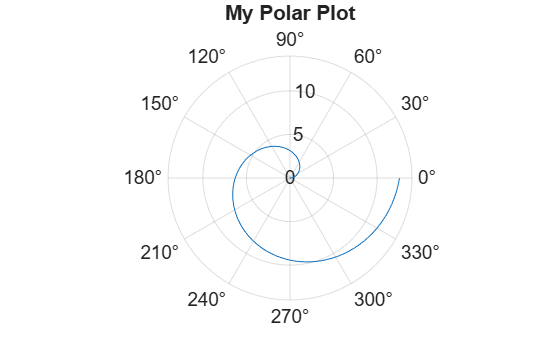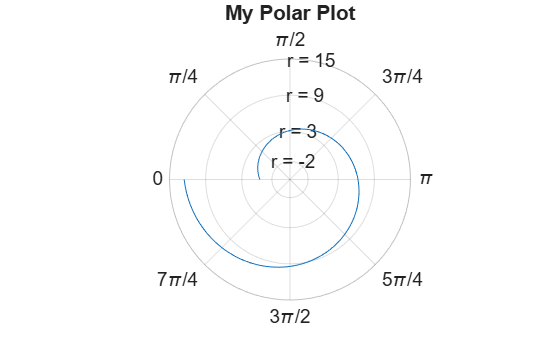Documentation

## Customize Polar Axes

You can modify certain aspects of polar axes in order to make the chart more readable. For example, you can change the grid line locations and associated labels. You also can change the grid line colors and label font size.

### Create Polar Plot

Plot a line in polar coordinates and add a title.

```theta = linspace(0,2*pi); rho = 2*theta; figure polarplot(theta,rho) title('My Polar Plot')```### Customize Polar Axes Using Properties

When you create a polar plot, MATLAB creates a `PolarAxes` object. `PolarAxes` objects have properties that you can use to customize the appearance of the polar axes, such as the font size, color, or ticks. For a full list, see PolarAxes Properties.

Access the PolarAxes object using the `gca` function, such as `pax = gca`. Then, use `pax` with dot notation to set properties, such as `pax.FontSize = 14`.

`pax = gca`
```pax = PolarAxes (My Polar Plot) with properties: ThetaLim: [0 360] RLim: [0 14] ThetaAxisUnits: 'degrees' ThetaDir: 'counterclockwise' ThetaZeroLocation: 'right' Show all properties ```
`pax.FontSize = 14;`### theta-Axis Tick Values

Display lines along the theta-axis every 45 degrees. Specify the locations as a vector of increasing values.

`thetaticks(0:45:315)`Display the theta-axis values in radians instead of degrees by setting the `ThetaAxisUnits` property.

```pax = gca; pax.ThetaAxisUnits = 'radians';```Modify the theta-axis so that it increases in a clockwise direction. Also, rotate the theta-axis so that the zero reference angle is on the left side.

```pax = gca; pax.ThetaDir = 'clockwise'; pax.ThetaZeroLocation = 'left';```### r-Axis Limits, Tick Values, and Labels

Change the limits of the r-axis so that the values range from -5 to 15. Display lines at the values -2, 3, 9, and 15. Then, change the labels that appear next to each line. Specify the labels as a cell array of character vectors.

```rlim([-5 15]) rticks([-2 3 9 15]) rticklabels({'r = -2','r = 3','r = 9','r = 15'})```### Grid Line and Label Colors

Use different colors for the theta-axis and r-axis grid lines and associated labels by setting the `ThetaColor` and `RColor` properties. Change the width of the grid lines by setting the `LineWidth` property.

Specify the colors using either a character vector of a color name, such as `'blue'`, or an RGB triplet. An RGB triplet is a three-element row vector whose elements specify the intensities of the red, green, and blue components of the color. The intensities must be in the range [0,1], for example, [0.4 0.6 0.7].

```pax = gca; pax.ThetaColor = 'blue'; pax.RColor = [0 .5 0];```Change the color of all the grid lines without affecting the labels by setting the `GridColor` property.

`pax.GridColor = 'red';`When you specify the `GridColor` property, the `ThetaColor` and `RColor` properties no longer affect the grid lines. If you want the `ThetaColor` and `RColor` properties to affect the grid lines, then set the `GridColorMode` property back to `'auto'`.